## Thursday, December 4, 2014

### Update to locate.yeti: REML and exact likelihood methods

I just posted code for a new version of the function `locate.yeti`. This function can be used to place recently extinct, missing, or cryptic taxa into an ultrametric phylogeny based on continuous character data.

The main updates are as follows:

(1) The function now includes a REML method which uses the contrasts algorithm of Felsenstein (1985). Note that this option is currently under development.

(2) The function also now includes exact likelihood calculation, rather then the approximate method that used orthogonalization of the original data based on the backbone tree. This obviously slows down computation, and I implemented it primarily to compare to the REML method (which does not need to use orthogonalization). It seems to not be prohibitively slow for relatively modest sized trees (say, 100 taxa or so).

Here's a quick demo.

First, simulate tree & data:

``````library(phytools)
``````
``````## Loading required package: ape
``````
``````library(phangorn)
``````
``````##
## Attaching package: 'phangorn'
##
## The following object is masked from 'package:ape':
##
##     as.prop.part
``````
``````## simulate tree & data
N<-50 ## taxa in base tree
m<-10 ## number of continuous characters
## simulate tree
tt<-tree<-pbtree(n=N+1,tip.label=sample(c(paste("t",1:N,sep=""),"Yeti")))
## generate a covariance matrix for simulation
L<-matrix(rnorm(n=m*m),m,m)
L[upper.tri(L,diag=FALSE)]<-0
L<-L-diag(diag(L))+abs(diag(diag(L)))
V<-L%*%t(L)
X<-sim.corrs(tree,vcv=V)
tree<-drop.tip(tree,"Yeti")
## visualize trees
par(mfrow=c(1,2))
plotTree(tt,mar=c(0.1,0.1,4.1,0.1),fsize=0.8)
title("tree with Yeti")
plotTree(tree,mar=c(0.1,0.1,4.1,0.1),direction="leftwards",fsize=0.8)
title("tree without Yeti")
``````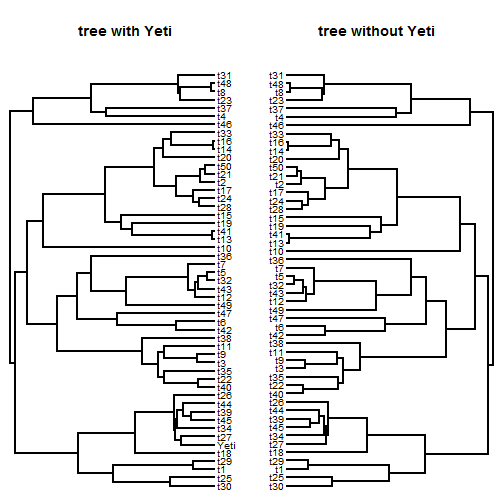OK, now let's estimate using the ML method with & without rotation:

``````## without rotation
mltree<-locate.yeti(tree,X,plot=TRUE,search="exhaustive",rotate=FALSE)
``````
``````## Optimizing the phylogenetic position of Yeti using ML. Please wait....
``````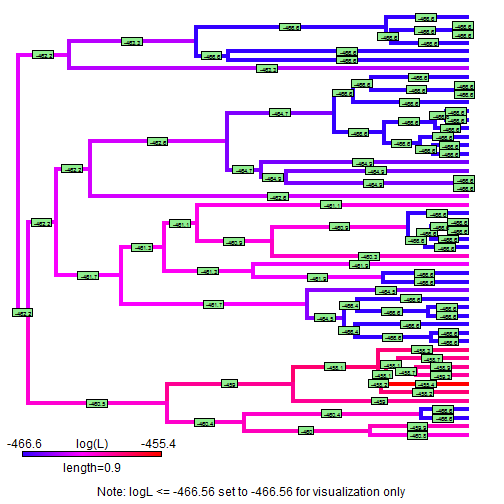``````## Done.
``````
``````mltree.rotate<-locate.yeti(tree,X,plot=TRUE,search="exhaustive")
``````
``````## Optimizing the phylogenetic position of Yeti using ML. Please wait....
``````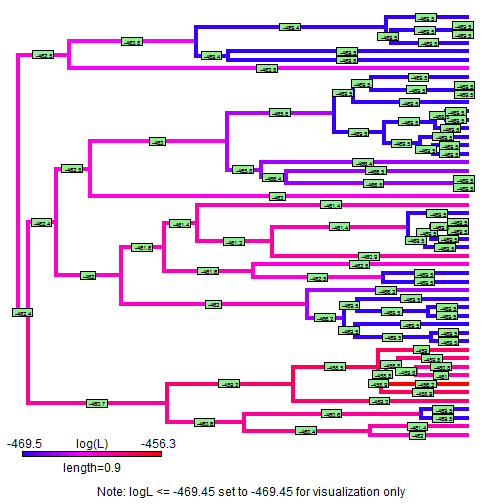``````## Done.
``````
``````mltree\$logL
``````
``````##  -455.4
``````
``````mltree.rotate\$logL
``````
``````##  -456.3
``````
``````RF.dist(mltree,mltree.rotate)
``````
``````##  0
``````

Hopefully, we get more or less the same tree in both cases!

Now, let's try the REML method. We can compare our results to the true tree and to our ML tree from above:

``````remltree<-locate.yeti(tree,X,method="REML",plot=TRUE,search="exhaustive")
``````
``````## ---------------------------------------------------------------
## | **Warning: method="REML" has not been thoroughly tested.    |
## |   Use with caution.**                                       |
## ---------------------------------------------------------------
##
## Optimizing the phylogenetic position of Yeti using REML. Please wait....
``````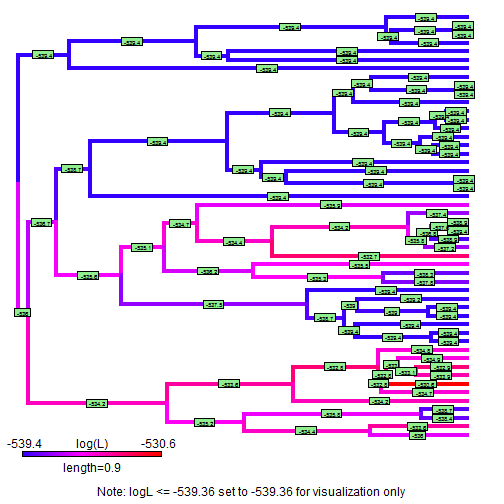``````## Done.
``````
``````RF.dist(tt,remltree)
``````
``````##  4
``````
``````RF.dist(mltree,remltree)
``````
``````##  0
``````

In addition to the RF distance, let's compute the patristic distances on the ML and REML trees. Note that it doesn't mean much that most of these are precisely the same - these are distances that don't involve the unknown tip. We should instead compare the very few outliers that involve distances from our unknown tip to other taxa in the tree:

``````par(mfrow=c(1,2))
plot(cophenetic(tt)[tt\$tip.label,tt\$tip.label],
cophenetic(mltree)[tt\$tip.label,tt\$tip.label],
xlab="true distances",ylab="ML tree distances")
plot(cophenetic(tt)[tt\$tip.label,tt\$tip.label],
cophenetic(remltree)[tt\$tip.label,tt\$tip.label],
xlab="true distances",ylab="REML tree distances")
``````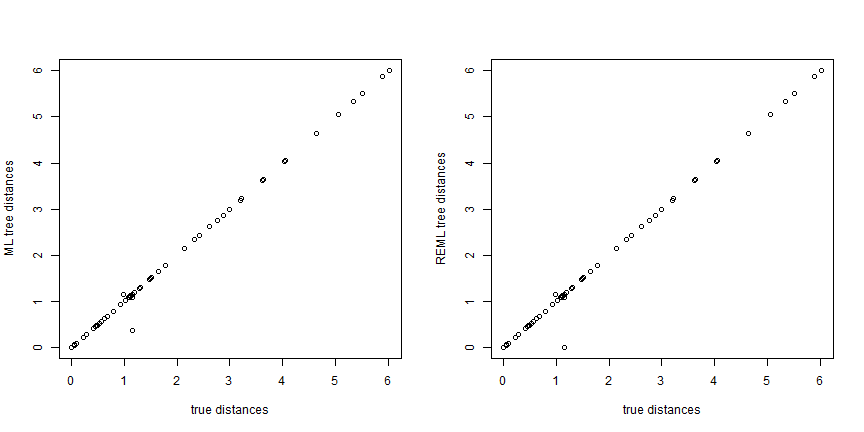OK, well, that's all I have. This update, along with the new phytools function `fitPagel`, is in a new non-CRAN phytools version (phytools 0.4-40) that I recently posted. Let me know if you run into any difficulties with either function.